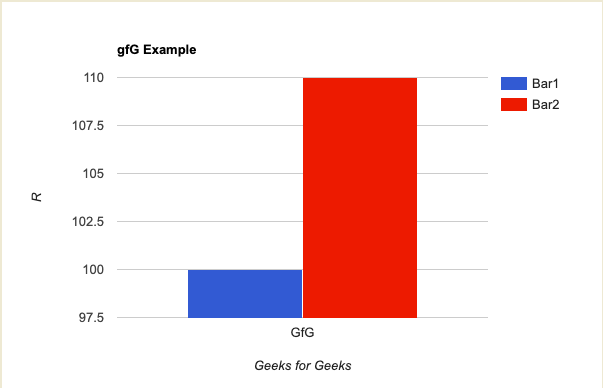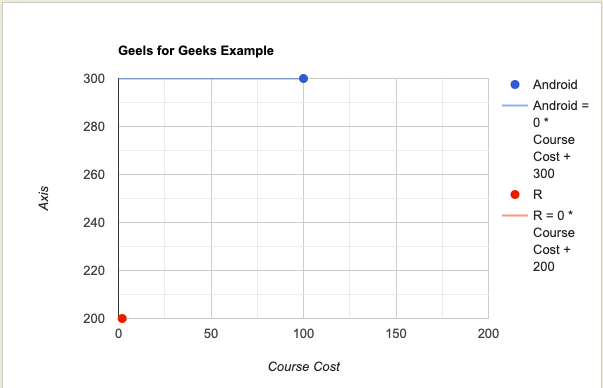# Tidycharts Package in R

• Last Updated : 01 May, 2022

In this article, we will be discussing the Tidycharts with their examples in the R programming language.

## R Tidycharts

• Tidycharts in R is an attractive visualization toolkit that makes plots that adhere to or follow “International Business Communication Standards” (IBCS).
• A little background about IBCS – In other words, IBCS has some plot requirements, such as unified bar widths, color combinations, and chart size.
• Tidycharts in R have a vital feature that will assist you in merging several charts with a clear picture.

## Installation

The Tidycharts library will be installed in R as the first step in this tutorial. Run the code below to install and load the library into R.

## R

 `# Install the package first``install.packages``(``"tidycharts"``)`` ` `# Then load the package``library``(tidycharts)`

## Tidycharts’ Bar Charts:

To make a bar chart in R using Tidycharts, we must first create a dataframe from which we can display a cohesive and visually pleasing bar chart.

To visualize the plot, you must use the Tidycharts library’s bar chart function.

## R

 `# You can create a bar chart like this``my_bar_chart < - ``bar_chart``(data, data\$city_names,``                           ``c``(``"Geeks for Geeks"``, ``"Courses"``),``                           ``c``(``"Geeks for Geeks"``, ``"Courses"``))``> my_bar_chart`## Using Tidycharts create a scatter plot:

When it comes to showing the relationship between two variables, scatter plots in R are the go-to charts. The data points are represented by the dots in the graph below. We attempted to depict the link between petal length and petal width for all of the species in the dataset using this scatter plot. This graphic is useful for viewing two numerical variables.

## R

 `# Create A Scatter Plot``my_scatter_plot < - ``scatter_plot``(iris, iris\$Petal.Length,``                                 ``iris\$Petal.Width, iris\$Species, ``                                 ``100, 200, ``c``(``"Course Cost"``, ``"R"``),``                                 ``c``(``"Course Cost"``, ``"Android"``),``                                 ``"Legend"``)`` ` `# View plot``gfg_scatter_plot`My Personal Notes arrow_drop_up